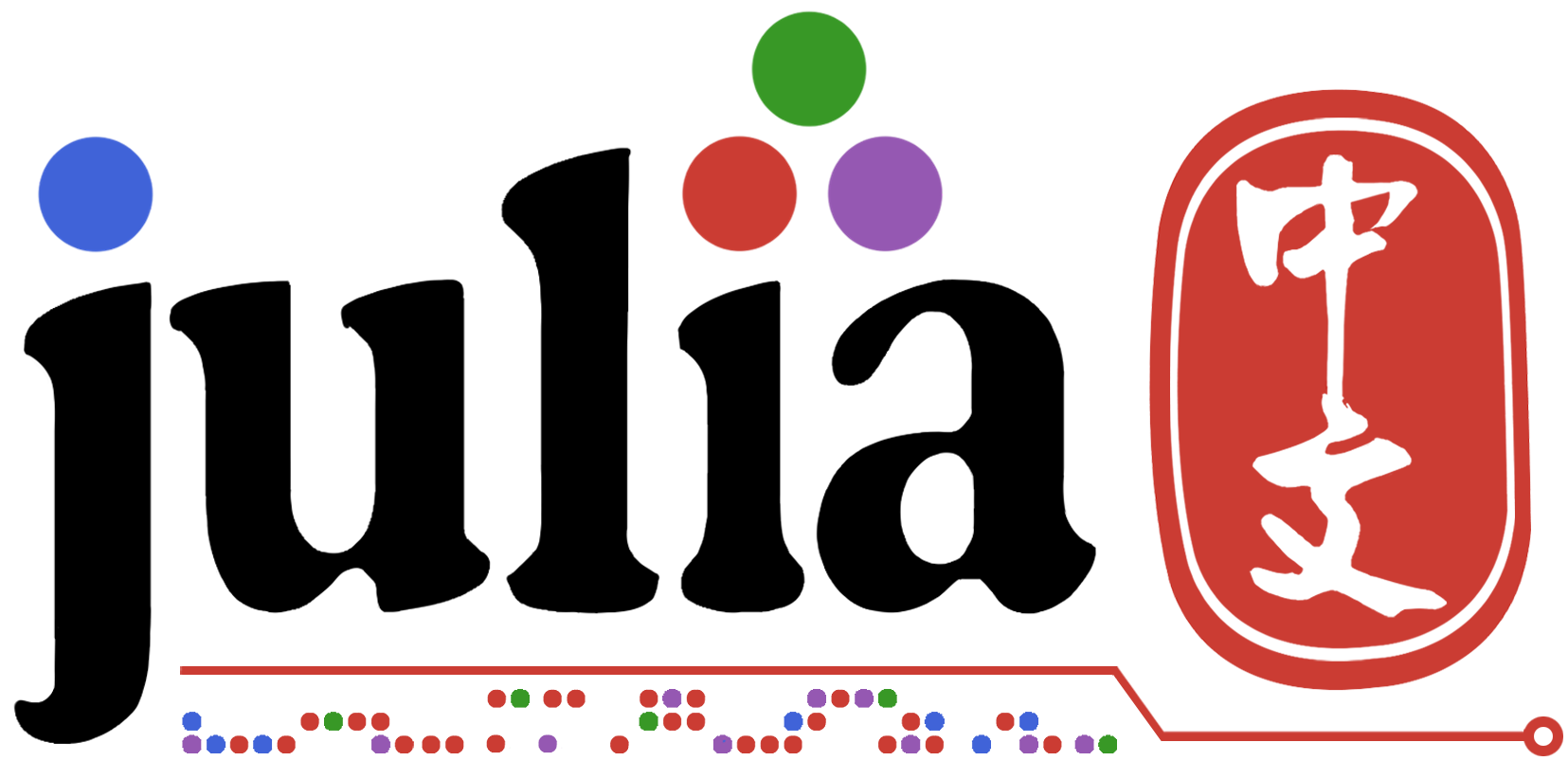# 求问关于PDE积分

``````using Flux
println("Integro Differential equation tests")
using DiffEqFlux
println("Starting Soon!")
using ModelingToolkit
using DiffEqBase
using Test, NeuralPDE
println("Starting Soon!")
using GalacticOptim
using Optim
using QuasiMonteCarlo
using SciMLBase
import ModelingToolkit: Interval, infimum, supremum
using DomainSets, Plots

using Random
Random.seed!(100)

cb = function (p,l)
println("Current loss is: \$l")
return false
end
``````
``````#simple multidimensitonal integral test
println("simple multidimensitonal integral test")

@parameters x,y
@variables u(..)
Dx = Differential(x)
Dy = Differential(y)
Ix = Integral((x,y) in DomainSets.UnitSquare())
eq = Ix(u(x,y)) ~ 1/3
bcs = [u(0., 0.) ~ 1, Dx(u(x,y)) ~ -2*x , Dy(u(x ,y)) ~ -2*y ]
domains = [x ∈ Interval(0.0,1.00), y ∈ Interval(0.0,1.00)]
chain = Chain(Dense(2,15,Flux.σ),Dense(15,1))
initθ = Float64.(DiffEqFlux.initial_params(chain))
strategy_ = NeuralPDE.GridTraining(0.1)
discretization = NeuralPDE.PhysicsInformedNN(chain,
strategy_;
init_params = nothing,
phi = nothing,
derivative = nothing,
)
@named pde_system = PDESystem(eq,bcs,domains,[x,y],[u(x, y)])
prob = NeuralPDE.discretize(pde_system,discretization)
res = GalacticOptim.solve(prob, BFGS(); cb = cb, maxiters=100)
phi = discretization.phi

xs = 0.00:0.01:1.00
ys = 0.00:0.01:1.00

u_real = collect(1 - x^2 - y^2 for y in ys, x in xs);
u_predict = collect(Array(phi([x,y], res.minimizer)) for y in ys, x in xs);
@test Flux.mse(u_real, u_predict) < 0.001

error_ = u_predict .- u_real
p1 = plot(xs,ys,u_real,linetype=:contourf,label = "analytic")
p2 = plot(xs,ys,u_predict,linetype=:contourf,label = "predict")
p3 = plot(xs,ys,error_,linetype=:contourf,label = "error")
plot(p1,p2,p3)
``````